Compile

You might want to download a related package posted on MathSource.  The package is posted at
http://library.wolfram.com/database/MathSource/555/

Examples of compiled functions that take an arbitrary length vector and an arbitrary dimension matrix

Using Compile[{{x1,t1,n1},...},expr] indicates (x1) is a rank (n1) tensor where each element has the type (t1).  A vector is a rank 1 tensor,and a matrix is a rank 2 tensor.  A function (magnitude) is defined below which finds the magnitude of an arbitrary length vector.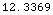Using Compile[{{x, _Real, 2}}, ...] indicates (x) is a matrix of real numbers.  The matrix can have any dimensions.  As an example a CompiledFunction (func) is defined below.  The function (func) takes two matrices of real numbers named (a) and (p) and computes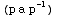.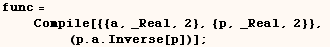A demonstration of (func) is given below.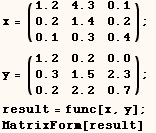How to make a compiled functions that run fast.

In version 3.0 Compile returns an expression with the form CompiledFunction[args, nregs, instr, func].  In version 4.0 Compile returns CompiledFunction[args, argregs, nregs, instr, func].  Either way the second from the last argument is (instr), a list of compiled function instructions.  Hence if (func) is a function defined using Compile func[[-2]] will return the list of instructions.  The compiled function instructions include low level operations such as 'store a real number in a particular register'.  You don't need to know what each instruction does, but you want make sure the instructions don't include something with the form Function[args, expr].  Any instructions with this form will probably cause the function to run much slower than it should because standard Mathematica evaluation instead of compiled evaluation will be used.

There are several reasons why the compiled function instructions would include the form Function[args,expr], and all reasons I know of are demonstrated below.

An important undocumented feature in Version 4

Version 4.0 has an undocumented feature that will post useful messages when a function is defined using Compile and a portion of the function can't be computed with compiled evaluation.  Evaluating the next cell will enable the undocumented feature.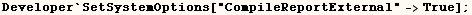Once the feature above is enabled (using Mathematica Version 4) a message is displayed after the next cell is evaluated.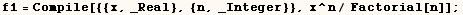(1)  Only a subset of kernel functions can be used in compiled evaluation.

One possible reason for having Function[args,expr] in the instructions is that (expr) may use one or more kernel functions that can't be represented as a low level op-code instruction (a list of integers).  As a rule of thumb functions that have a simple translation into C can be converted to op-code.  This is only a rule of thumb because Factorial has a simple translation into C, but can't be represented in op-code.  In fact that is why the compiled function instructions for (f1) below includes Function[{x,n},n!].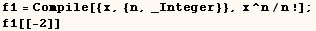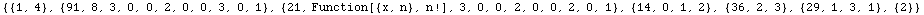The function (f2) in the next cell does the same thing as (f1) in the previous cell, and will evaluate using compiled evaluation because the compiled function instructions don't include the form Function[args,instr].An alphabetical list of kernel features that can be converted to op-code can be found by searching for Compile at: http://www.wolfram.com/support/Kernel/Symbols/.   The same symbols are sorted below by category below.

Math functions and constants

Plus, Times, Power, Sqrt,
Divide, Minus, Subtract, Quotient,
Sign, Abs, Arg, Re, Im, Conjugate,
Floor, Ceiling, Round, Mod, Min, Max, Log, Exp,
Sin, Cos, Tan, ArcSin, ArcCos, ArcTan,
Csc, Sec, Cot, ArcCsc, ArcSec, ArcCot,
Sinh, Cosh, Tanh, ArcSinh, ArcCosh, ArcTanh,
Csch, Sech, Coth, ArcCsch, ArcSech, ArcCoth,
N, Chop, Random, IntegerDigits, Product, Sum, EulerE
E, Pi, Degree, GoldenRatio, Catalan

Compile in Version 4 and later supports the following:
UnitStep, Khinchin, Glaisher, BitAnd, BitOr, BitXor, BitNot

Vector, matrix and tensor mathematics

List, Det, Inverse, Dot, DiagonalMatrix, IdentityMatrix,
Transpose, Dimensions, TensorRank

Boolean related features

True, False, Not, And, Or, Xor, Equal, Unequal,
Greater, GreaterEqual, Less, LessEqual, Equal, Unequal, SameQ, UnsameQ,
EvenQ, OddQ, Positive, Negative, NonNegative, FreeQ, MemberQ, OrderedQ,
VectorQ, MatrixQ

Building and manipulating expressions and lists

Set, List, CompoundExpression, Length,
Array, Table, Range, Count, Select, Cases, DeleteCases,
Position, ReplacePart, Join, Intersection, Complement, Union,
Append, AppendTo, Prepend, PrependTo,
Part, Take, Insert, Delete, Drop, Rest, First, Last,
Flatten, Sort, Reverse, RotateLeft, RotateRight, Partition, Permutations

Functional programming

Apply, Map, MapAll, MapAt, MapIndexed, MapThread,
Scan, FixedPoint, FixedPointList, Nest, NestList,
Fold, FoldList, Array, Outer, ComposeList, Function, Slot, SlotSequence

Scoping and procedural programming

Module, With, Block, Do, While, For,
Set, If, Switch, Which, Implies,
Break, Continue, Catch, Throw, Goto, Label, Return,
Increment, Decrement, PreIncrement, PreDecrement,
AddTo, SubtractFrom, TimesBy, DivideBy, Null, \$Failed

(2)  Compiled evaluation can't use global variables.

In the next cell a compiled function uses a global variable (width).  Later we see that the function works, but timing tests will show that it's no faster than if the function was defined without using Compile.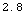The next cell defines the same function as the previous example and takes the global variable as an argument. This is the way global variables should be used in a compiled function.The next cell shows what happens if we try to set the value of a global variable inside a compiled function.  Here again the function works, but timing tests show that the function is no faster than the same function defined without using Compile.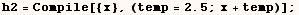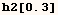In the last example the value of (temp) was set inside Compile.  When something like this is needed Block, Module, or With should be used to make (temp) a local variable.  Compiled functions are defined below that do the same thing as the last example using Block, Module, With and they all use compiled evaluation which runs much faster.  Besides the advantage in speed the approach below ensures that evaluating say (ha[1.5], hb[1.5], hc[1.5]) will not change the value (temp) might have outside the compiled function.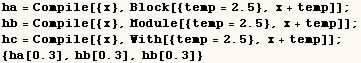(3)  Compiled evaluation can't work with patterns.

The function in the next cell takes a list of real numbers, and determines if (0.5) is in the list.  This function will use compiled evaluation.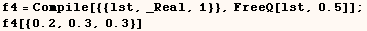The function in the next cell also takes a list of real numbers, and determines if the list is free of negative numbers.  This function works, but timing tests will show that it's no faster than the same function defined without using Compile.  The problem in this case is that the compiled function uses the pattern (_?Negative).  Compiled evaluation isn't used if the second argument in Compile includes patterns of any kind.  When faced with a problem like this we should use an algorithm that doesn't require patterns, or define a function without using Compile.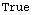The next cell shows one way the function from the last example can be written to avoid the use of patterns.  This version will use compiled evaluation.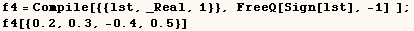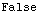(4)  Local variables in compiled evaluation must always have the same type.

The next cell defines a function which uses a local variable (temp) where temp starts with an integer value.  Later in the function (temp) is changed to a real number.  In this case the function works, but timing tests will show it's no faster than the same function defined without using Compile.  Local variables used in a compiled function should always have the same type (Real, Integer, Complex, True|False) to ensure compiled evaluation is used.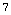(5)  A compiled function can't change the value of it's argument.

The next cell shows an attempt to define a function.  If you try to use this function you will see it doesn't work.  The problem here is that the function tries to change the value of it's argument, but a compiled function can't change the value of it's argument for the same reason we can't evaluate (3=5).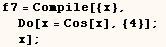Some times we want to use an algorithm that starts with some value and replaces it with another value.  If we want to do this in a compiled function we should initialize a local variable to the value of the functions argument, and then change the value of the local variable.  The function in the next cell does this and uses compiled evaluation.(6)  Compiled evaluation can't work with all possible list structures.

The only list structures compiled evaluation can work with are vectors, matrices, and other tensors with (rank<101).  The function defined in the next cell returns a list structure that doesn't meet these restrictions so compiled evaluation can't be used.  In a case like this there is no advantage to using Compile to define the function.Created by Mathematica  (May 16, 2004)

Back to Ted’s Tricks index page